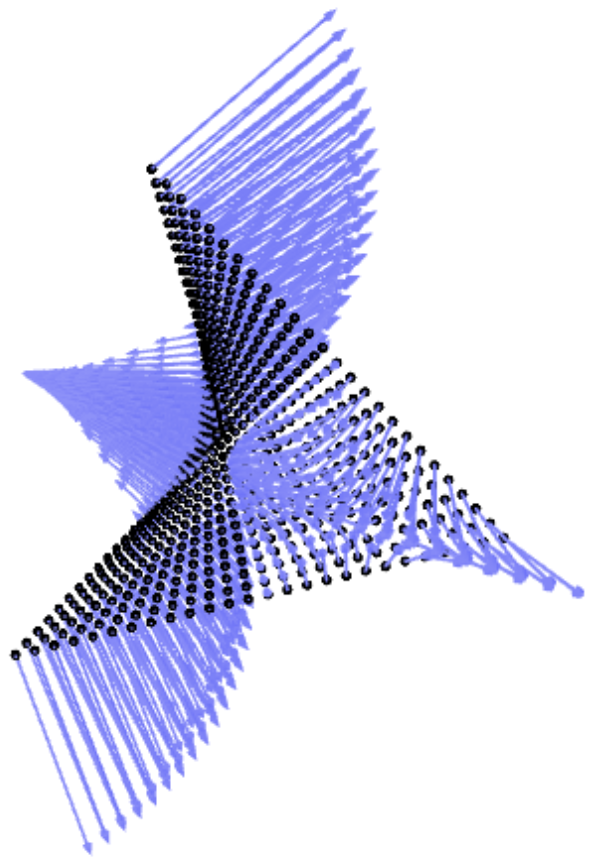# Minimality of tensors of fixed multilinear rank

Alexander Heaton, Khazhgali Kozhasov, and Lorenzo Venturello: Minimality of tensors of fixed multilinear rank
In: Linear and multilinear algebra, Vol. not yet known, pp. not yet known

In this article, we discover a geometric property of the space of tensors of fixed multilinear (Tucker) rank. Namely, we show that real tensors of fixed multilinear rank form a minimal submanifold of the Euclidean space of all tensors of given format endowed with the Frobenius inner product.

Here, we present a computation which verifies a (very) special case of our main theorem. Namely, we compute the mean curvature vector field of a submanifold of Euclidean space, showing that it is everywhere vanishing. We also compute the mean curvature vector field of a related submanifold of interest in statistics, formed by slicing the minimal sub manifold with an affine hyperplane. Since this submanifold is no longer minimal, we visualize its mean curvature vector field, shown below.The Jupiter file making these computations can be seen by clicking the link below.

The Jupyter notebook can be downloaded `here`.

Project page created: 25/11/2020

Code contributors: Alexander Heaton

Jupyter Notebook written by: Alexander Heaton, 25/11/2020

Project contributors: Alexander Heaton

Software used: SageMath 9.1.